Download the Jupyter Notebook for this section: post_estimation.ipynb

# Post-Estimation Tutorial¶

:

%matplotlib inline

import pyblp
import numpy as np
import pandas as pd
import matplotlib.pyplot as plt

pyblp.options.digits = 2
pyblp.options.verbose = False
pyblp.__version__

:

'0.11.0'


This tutorial covers several features of pyblp which are available after estimation including:

1. Calculating elasticities and diversion ratios.

2. Calculating marginal costs and markups.

3. Computing the effects of mergers: prices, shares, and HHI.

4. Using a parametric bootstrap to estimate standard errors.

5. Estimating optimal instruments.

## Problem Results¶

As in the fake cereal tutorial, we’ll first solve the fake cereal problem from Nevo (2000). We load the fake data and estimate the model as in the previous tutorial. We output the setup of the model to confirm we have correctly configured the Problem

:

product_data = pd.read_csv(pyblp.data.NEVO_PRODUCTS_LOCATION)
product_formulations = (
pyblp.Formulation('0 + prices', absorb='C(product_ids)'),
pyblp.Formulation('1 + prices + sugar + mushy')
)
agent_formulation = pyblp.Formulation('0 + income + income_squared + age + child')
problem = pyblp.Problem(product_formulations, product_data, agent_formulation, agent_data)
problem

:

Dimensions:
=================================================
T    N     F    I     K1    K2    D    MD    ED
---  ----  ---  ----  ----  ----  ---  ----  ----
94   2256   5   1880   1     4     4    20    1
=================================================

Formulations:
===================================================================
Column Indices:           0           1           2      3
-----------------------------  ------  --------------  -----  -----
X1: Linear Characteristics    prices
X2: Nonlinear Characteristics    1         prices      sugar  mushy
d: Demographics         income  income_squared   age   child
===================================================================


We’ll solve the problem in the same way as before. The Problem.solve method returns a ProblemResults class, which displays basic estimation results. The results that are displayed are simply formatted information extracted from various class attributes such as ProblemResults.sigma and ProblemResults.sigma_se.

:

initial_sigma = np.diag([0.3302, 2.4526, 0.0163, 0.2441])
initial_pi = [
[ 5.4819,  0,      0.2037,  0     ],
[15.8935, -1.2000, 0,       2.6342],
[-0.2506,  0,      0.0511,  0     ],
[ 1.2650,  0,     -0.8091,  0     ]
]
results = problem.solve(
initial_sigma,
initial_pi,
optimization=pyblp.Optimization('bfgs', {'gtol': 1e-5}),
method='1s'
)
results

:

Problem Results Summary:
=======================================================================================================
GMM   Objective  Gradient      Hessian         Hessian     Clipped  Weighting Matrix  Covariance Matrix
Step    Value      Norm    Min Eigenvalue  Max Eigenvalue  Shares   Condition Number  Condition Number
----  ---------  --------  --------------  --------------  -------  ----------------  -----------------
1    +4.6E+00   +6.9E-06     +2.7E-05        +1.6E+04        0         +6.9E+07          +8.4E+08
=======================================================================================================

Cumulative Statistics:
===========================================================================
Computation  Optimizer  Optimization   Objective   Fixed Point  Contraction
Time      Converged   Iterations   Evaluations  Iterations   Evaluations
-----------  ---------  ------------  -----------  -----------  -----------
00:01:36       Yes          51           57          45483       141195
===========================================================================

Nonlinear Coefficient Estimates (Robust SEs in Parentheses):
=====================================================================================================================
Sigma:      1         prices      sugar       mushy     |   Pi:      income    income_squared     age        child
------  ----------  ----------  ----------  ----------  |  ------  ----------  --------------  ----------  ----------
1      +5.6E-01                                       |    1      +2.3E+00      +0.0E+00      +1.3E+00    +0.0E+00
(+1.6E-01)                                      |          (+1.2E+00)                  (+6.3E-01)
|
prices   +0.0E+00    +3.3E+00                           |  prices   +5.9E+02      -3.0E+01      +0.0E+00    +1.1E+01
(+1.3E+00)                          |          (+2.7E+02)    (+1.4E+01)                (+4.1E+00)
|
sugar    +0.0E+00    +0.0E+00    -5.8E-03               |  sugar    -3.8E-01      +0.0E+00      +5.2E-02    +0.0E+00
(+1.4E-02)              |          (+1.2E-01)                  (+2.6E-02)
|
mushy    +0.0E+00    +0.0E+00    +0.0E+00    +9.3E-02   |  mushy    +7.5E-01      +0.0E+00      -1.4E+00    +0.0E+00
(+1.9E-01)  |          (+8.0E-01)                  (+6.7E-01)
=====================================================================================================================

Beta Estimates (Robust SEs in Parentheses):
==========
prices
----------
-6.3E+01
(+1.5E+01)
==========


Additional post-estimation outputs can be computed with ProblemResults methods.

## Elasticities and Diversion Ratios¶

We can estimate elasticities, $$\varepsilon$$, and diversion ratios, $$\mathscr{D}$$, with ProblemResults.compute_elasticities and ProblemResults.compute_diversion_ratios.

As a reminder, elasticities in each market are

(1)$\varepsilon_{jk} = \frac{x_k}{s_j}\frac{\partial s_j}{\partial x_k}.$

Diversion ratios are

(2)$\mathscr{D}_{jk} = -\frac{\partial s_k}{\partial x_j} \Big/ \frac{\partial s_j}{\partial x_j}.$

Following Conlon and Mortimer (2018), we report the diversion to the outside good $$D_{j0}$$ on the diagonal instead of $$D_{jj}=-1$$.

:

elasticities = results.compute_elasticities()
diversions = results.compute_diversion_ratios()


Post-estimation outputs are computed for each market and stacked. We’ll use matplotlib functions to display the matrices associated with a single market.

:

single_market = product_data['market_ids'] == 'C01Q1'
plt.colorbar(plt.matshow(elasticities[single_market]));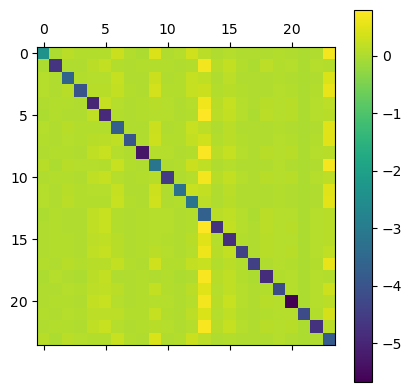:

plt.colorbar(plt.matshow(diversions[single_market]));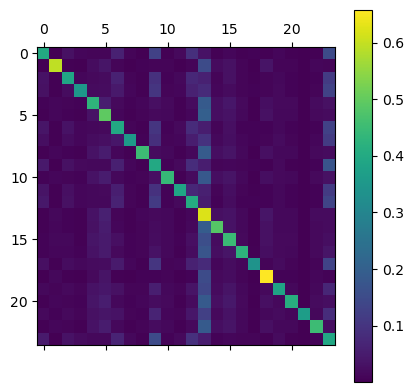The diagonal of the first image consists of own elasticities and the diagonal of the second image consists of diversion ratios to the outside good. As one might expect, own price elasticities are large and negative while cross-price elasticities are positive but much smaller.

Elasticities and diversion ratios can be computed with respect to variables other than prices with the name argument of ProblemResults.compute_elasticities and ProblemResults.compute_diversion_ratios. Additionally, ProblemResults.compute_long_run_diversion_ratios can be used to used to understand substitution when products are eliminated from the choice set.

The convenience methods ProblemResults.extract_diagonals and ProblemResults.extract_diagonal_means can be used to extract information about own elasticities of demand from elasticity matrices.

:

means = results.extract_diagonal_means(elasticities)


An alternative to summarizing full elasticity matrices is to use ProblemResults.compute_aggregate_elasticities to estimate aggregate elasticities of demand, $$E$$, in each market, which reflect the change in total sales under a proportional sales tax of some factor.

:

aggregates = results.compute_aggregate_elasticities(factor=0.1)


Since demand for an entire product category is generally less elastic than the average elasticity of individual products, mean own elasticities are generally larger in magnitude than aggregate elasticities.

:

plt.hist(
[means.flatten(), aggregates.flatten()],
color=['red', 'blue'],
bins=50
);
plt.legend(['Mean Own Elasticities', 'Aggregate Elasticities']);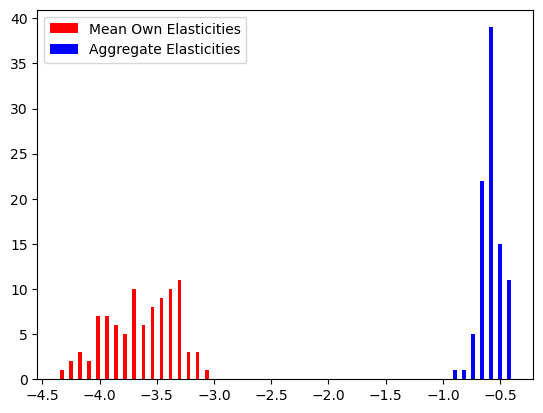## Marginal Costs and Markups¶

To compute marginal costs, $$c$$, the product_data passed to Problem must have had a firm_ids field. Since we included firm IDs when configuring the problem, we can use ProblemResults.compute_costs.

:

costs = results.compute_costs()
plt.hist(costs, bins=50);
plt.legend(["Marginal Costs"]);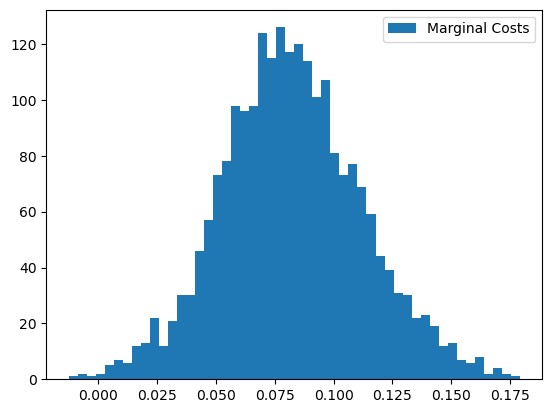Other methods that compute supply-side outputs often compute marginal costs themselves. For example, ProblemResults.compute_markups will compute marginal costs when estimating markups, $$\mathscr{M}$$, but computation can be sped up if we just use our pre-computed values.

:

markups = results.compute_markups(costs=costs)
plt.hist(markups, bins=50);
plt.legend(["Markups"]);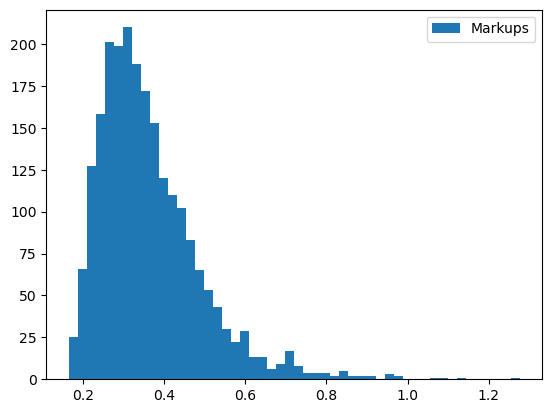## Mergers¶

Before computing post-merger outputs, we’ll supplement our pre-merger markups with some other outputs. We’ll compute Herfindahl-Hirschman Indices, $$\text{HHI}$$, with ProblemResults.compute_hhi; population-normalized gross expected profits, $$\pi$$, with ProblemResults.compute_profits; and population-normalized consumer surpluses, $$\text{CS}$$, with ProblemResults.compute_consumer_surpluses.

:

hhi = results.compute_hhi()
profits = results.compute_profits(costs=costs)
cs = results.compute_consumer_surpluses()


To compute post-merger outputs, we’ll create a new set of firm IDs that represent a merger of firms 2 and 1.

:

product_data['merger_ids'] = product_data['firm_ids'].replace(2, 1)


We can use ProblemResults.compute_approximate_prices or ProblemResults.compute_prices to estimate post-merger prices. The first method, which is discussed, for example, in Nevo (1997), assumes that shares and their price derivatives are unaffected by the merger. The second method does not make these assumptions and iterates over the $$\zeta$$-markup equation from Morrow and Skerlos (2011) to solve the full system of $$J_t$$ equations and $$J_t$$ unknowns in each market $$t$$. We’ll use the latter, since it is fast enough for this example problem.

:

changed_prices = results.compute_prices(
firm_ids=product_data['merger_ids'],
costs=costs
)


We’ll compute post-merger shares with ProblemResults.compute_shares.

:

changed_shares = results.compute_shares(changed_prices)


Post-merger prices and shares are used to compute other post-merger outputs. For example, $$\text{HHI}$$ increases.

:

changed_hhi = results.compute_hhi(
firm_ids=product_data['merger_ids'],
shares=changed_shares
)
plt.hist(changed_hhi - hhi, bins=50);
plt.legend(["HHI Changes"]);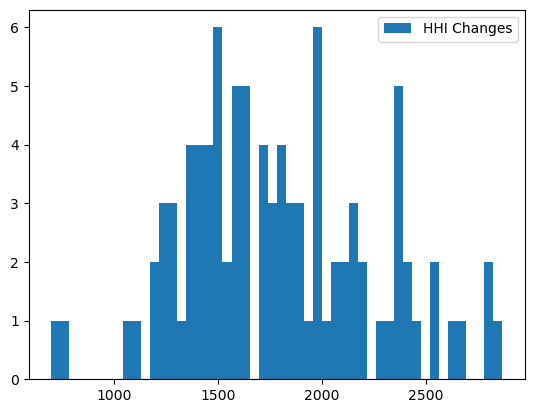Markups, $$\mathscr{M}$$, and profits, $$\pi$$, generally increase as well.

:

changed_markups = results.compute_markups(changed_prices, costs)
plt.hist(changed_markups - markups, bins=50);
plt.legend(["Markup Changes"]);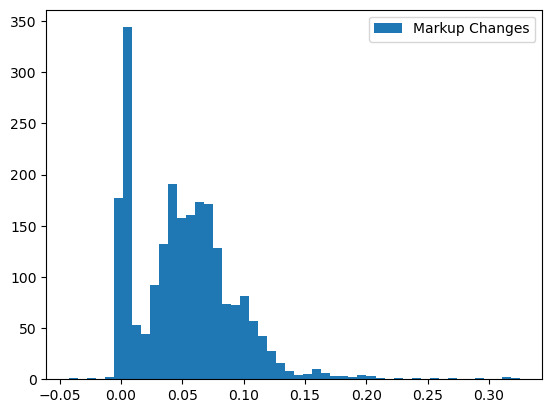:

changed_profits = results.compute_profits(changed_prices, changed_shares, costs)
plt.hist(changed_profits - profits, bins=50);
plt.legend(["Profit Changes"]);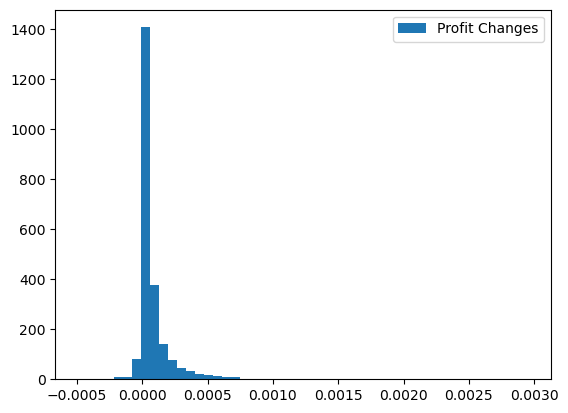On the other hand, consumer surpluses, $$\text{CS}$$, generally decrease.

:

changed_cs = results.compute_consumer_surpluses(changed_prices)
plt.hist(changed_cs - cs, bins=50);
plt.legend(["Consumer Surplus Changes"]);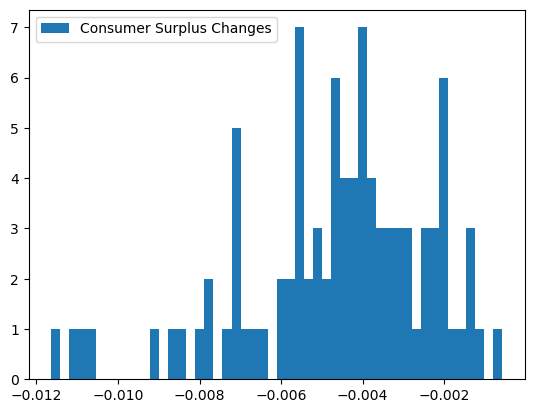## Bootstrapping Results¶

Post-estimation outputs can be informative, but they don’t mean much without a sense sample-to-sample variability. One way to estimate confidence intervals for post-estimation outputs is with a standard bootstrap procedure:

1. Construct a large number of bootstrap samples by sampling with replacement from the original product data.

2. Initialize and solve a Problem for each bootstrap sample.

3. Compute the desired post-estimation output for each bootstrapped ProblemResults and from the resulting empirical distribution, construct boostrap confidence intervals.

Although appealing because of its simplicity, the computational resources required for this procedure are often prohibitively expensive. Furthermore, human oversight of the optimization routine is often required to determine whether the routine ran into any problems and if it successfully converged. Human oversight of estimation for each bootstrapped problem is usually not feasible.

A more reasonable alternative is a parametric bootstrap procedure:

1. Construct a large number of draws from the estimated joint distribution of parameters.

2. Compute the implied mean utility, $$\delta$$, and shares, $$s$$, for each draw. If a supply side was estimated, also computed the implied marginal costs, $$c$$, and prices, $$p$$.

3. Compute the desired post-estimation output under each of these parametric bootstrap samples. Again, from the resulting empirical distribution, construct boostrap confidence intervals.

Compared to the standard bootstrap procedure, the parametric bootstrap requires far fewer computational resources, and is simple enough to not require human oversight of each bootstrap iteration. The primary complication to this procedure is that when supply is estimated, equilibrium prices and shares need to be computed for each parametric bootstrap sample by iterating over the $$\zeta$$-markup equation from Morrow and Skerlos (2011). Although nontrivial, this fixed point iteration problem is much less demanding than the full optimization routine required to solve the BLP problem from the start.

An empirical distribution of results computed according to this parametric bootstrap procedure can be created with the ProblemResults.bootstrap method, which returns a BootstrappedResults class that can be used just like ProblemResults to compute various post-estimation outputs. The difference is that BootstrappedResults methods return arrays with an extra first dimension, along which bootstrapped results are stacked.

We’ll construct 90% parametric bootstrap confidence intervals for estimated mean own elasticities in each market of the fake cereal problem. Usually, bootstrapped confidence intervals should be based on thousands of draws, but we’ll only use a few for the sake of speed in this example.

:

bootstrapped_results = results.bootstrap(draws=100, seed=0)
bootstrapped_results

:

Bootstrapped Results Summary:
======================
Computation  Bootstrap
Time        Draws
-----------  ---------
00:00:24       100
======================

:

bounds = np.percentile(
bootstrapped_results.extract_diagonal_means(
bootstrapped_results.compute_elasticities()
),
q=[10, 90],
axis=0
)
table = pd.DataFrame(index=problem.unique_market_ids, data={
'Lower Bound': bounds.flatten(),
'Mean Own Elasticity': aggregates.flatten(),
'Upper Bound': bounds.flatten()
})

:

Lower Bound Mean Own Elasticity Upper Bound
C01Q1 -4.31 -0.81 -3.88
C01Q2 -4.07 -0.69 -3.68
C03Q1 -3.71 -0.55 -3.20
C03Q2 -3.65 -0.60 -3.16
C04Q1 -3.31 -0.68 -2.97

## Optimal Instruments¶

Given a consistent estimate of $$\theta$$, we may want to compute the optimal instruments of Chamberlain (1987) and use them to re-solve the problem. Optimal instruments have been shown, for example, by Reynaert and Verboven (2014), to reduce bias, improve efficiency, and enhance stability of BLP estimates.

The ProblemResults.compute_optimal_instruments method computes the expected Jacobians that comprise the optimal instruments by integrating over the density of $$\xi$$ (and $$\omega$$ if a supply side was estimated). By default, the method approximates this integral by averaging over the Jacobian realizations computed under draws from the asymptotic normal distribution of the error terms. Since this process is computationally expensive and often doesn’t make much of a difference, we’ll use method='approximate' in this example to simply evaluate the Jacobians at the expected value of $$\xi$$, zero.

:

instrument_results = results.compute_optimal_instruments(method='approximate')
instrument_results

:

Optimal Instrument Results Summary:
=======================
Computation  Error Term
Time        Draws
-----------  ----------
00:00:01        1
=======================


We can use the OptimalInstrumentResults.to_problem method to re-create the fake cereal problem with the estimated optimal excluded instruments.

:

updated_problem = instrument_results.to_problem()
updated_problem

:

Dimensions:
=================================================
T    N     F    I     K1    K2    D    MD    ED
---  ----  ---  ----  ----  ----  ---  ----  ----
94   2256   5   1880   1     4     4    14    1
=================================================

Formulations:
===================================================================
Column Indices:           0           1           2      3
-----------------------------  ------  --------------  -----  -----
X1: Linear Characteristics    prices
X2: Nonlinear Characteristics    1         prices      sugar  mushy
d: Demographics         income  income_squared   age   child
===================================================================


We can solve this updated problem just like the original one. We’ll start at our consistent estimate of $$\theta$$.

:

updated_results = updated_problem.solve(
results.sigma,
results.pi,
optimization=pyblp.Optimization('bfgs', {'gtol': 1e-5}),
method='1s'
)
updated_results

:

Problem Results Summary:
=======================================================================================================
GMM   Objective  Gradient      Hessian         Hessian     Clipped  Weighting Matrix  Covariance Matrix
Step    Value      Norm    Min Eigenvalue  Max Eigenvalue  Shares   Condition Number  Condition Number
----  ---------  --------  --------------  --------------  -------  ----------------  -----------------
1    +8.0E-14   +3.0E-06     +1.6E-04        +2.9E+04        0         +7.8E+07          +1.8E+08
=======================================================================================================

Cumulative Statistics:
===========================================================================
Computation  Optimizer  Optimization   Objective   Fixed Point  Contraction
Time      Converged   Iterations   Evaluations  Iterations   Evaluations
-----------  ---------  ------------  -----------  -----------  -----------
00:01:47       Yes          42           50          45102       139676
===========================================================================

Nonlinear Coefficient Estimates (Robust SEs in Parentheses):
=====================================================================================================================
Sigma:      1         prices      sugar       mushy     |   Pi:      income    income_squared     age        child
------  ----------  ----------  ----------  ----------  |  ------  ----------  --------------  ----------  ----------
1      +2.1E-01                                       |    1      +6.0E+00      +0.0E+00      +1.6E-01    +0.0E+00
(+7.8E-02)                                      |          (+5.2E-01)                  (+2.0E-01)
|
prices   +0.0E+00    +3.0E+00                           |  prices   +9.8E+01      -5.6E+00      +0.0E+00    +4.1E+00
(+6.5E-01)                          |          (+8.6E+01)    (+4.5E+00)                (+2.2E+00)
|
sugar    +0.0E+00    +0.0E+00    +2.7E-02               |  sugar    -3.1E-01      +0.0E+00      +4.9E-02    +0.0E+00
(+7.2E-03)              |          (+3.5E-02)                  (+1.3E-02)
|
mushy    +0.0E+00    +0.0E+00    +0.0E+00    +3.0E-01   |  mushy    +9.7E-01      +0.0E+00      -5.4E-01    +0.0E+00
(+1.0E-01)  |          (+2.9E-01)                  (+1.8E-01)
=====================================================================================================================

Beta Estimates (Robust SEs in Parentheses):
==========
prices
----------
-3.1E+01
(+4.5E+00)
==========